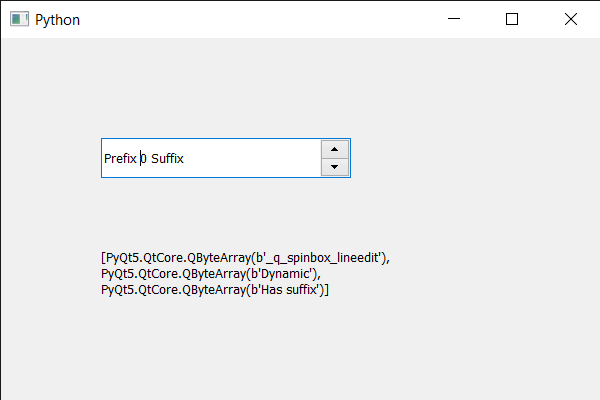Related Articles
PyQt5 QSpinBox – Getting all the properties names
• Last Updated : 19 May, 2020

In this article we will see how we can get all the properties name of the spin box that are added dynamically to it, properties are the information added by the developer to tell about the properties of the spin box, for example, if spin box has ability to loop the values, developer will add this property so that other developer can see this and get to know about the property. Properties are added using `setProperty` method.

In order to do this we use dynamicPropertyNames method

Syntax : spin_box.dynamicPropertyNames()

Argument : It takes no argument

Return : It returns list

Below is the implementation

 `# importing libraries ` `from` `PyQt5.QtWidgets ``import` `*`  `from` `PyQt5 ``import` `QtCore, QtGui ` `from` `PyQt5.QtGui ``import` `*`  `from` `PyQt5.QtCore ``import` `*`  `import` `sys ` ` `  ` `  `class` `Window(QMainWindow): ` ` `  `    ``def` `__init__(``self``): ` `        ``super``().__init__() ` ` `  `        ``# setting title ` `        ``self``.setWindowTitle(``"Python "``) ` ` `  `        ``# setting geometry ` `        ``self``.setGeometry(``100``, ``100``, ``600``, ``400``) ` ` `  `        ``# calling method ` `        ``self``.UiComponents() ` ` `  `        ``# showing all the widgets ` `        ``self``.show() ` ` `  `        ``# method for widgets ` `    ``def` `UiComponents(``self``): ` `        ``# creating spin box ` `        ``self``.spin ``=` `QSpinBox(``self``) ` ` `  `        ``# setting geometry to spin box ` `        ``self``.spin.setGeometry(``100``, ``100``, ``250``, ``40``) ` ` `  `        ``# setting range to the spin box ` `        ``self``.spin.setRange(``0``, ``999999``) ` ` `  `        ``# setting prefix to spin ` `        ``self``.spin.setPrefix(``"Prefix "``) ` ` `  `        ``# setting suffix to spin ` `        ``self``.spin.setSuffix(``" Suffix"``) ` ` `  `        ``# adding property to the spin box ` `        ``self``.spin.setProperty(``"Dynamic"``, ``1``) ` ` `  `        ``# adding property to the spin box ` `        ``self``.spin.setProperty(``"Has suffix"``, ``1``) ` ` `  `        ``# creating label ` `        ``self``.label ``=` `QLabel(``self``) ` ` `  `        ``# setting geometry to the label ` `        ``self``.label.setGeometry(``100``, ``200``, ``300``, ``70``) ` ` `  `        ``# making it multi line label ` `        ``self``.label.setWordWrap(``True``) ` ` `  `        ``# getting property names ` `        ``p_names ``=` `self``.spin.dynamicPropertyNames() ` ` `  `        ``# setting text to the label ` `        ``self``.label.setText(``str``(p_names)) ` ` `  `# create pyqt5 app ` `App ``=` `QApplication(sys.argv) ` ` `  `# create the instance of our Window ` `window ``=` `Window() ` `window.spin.setFocus() ` `# start the app ` `sys.exit(App.``exec``()) `

Output :Attention geek! Strengthen your foundations with the Python Programming Foundation Course and learn the basics.

To begin with, your interview preparations Enhance your Data Structures concepts with the Python DS Course.

My Personal Notes arrow_drop_up
Recommended Articles
Page :